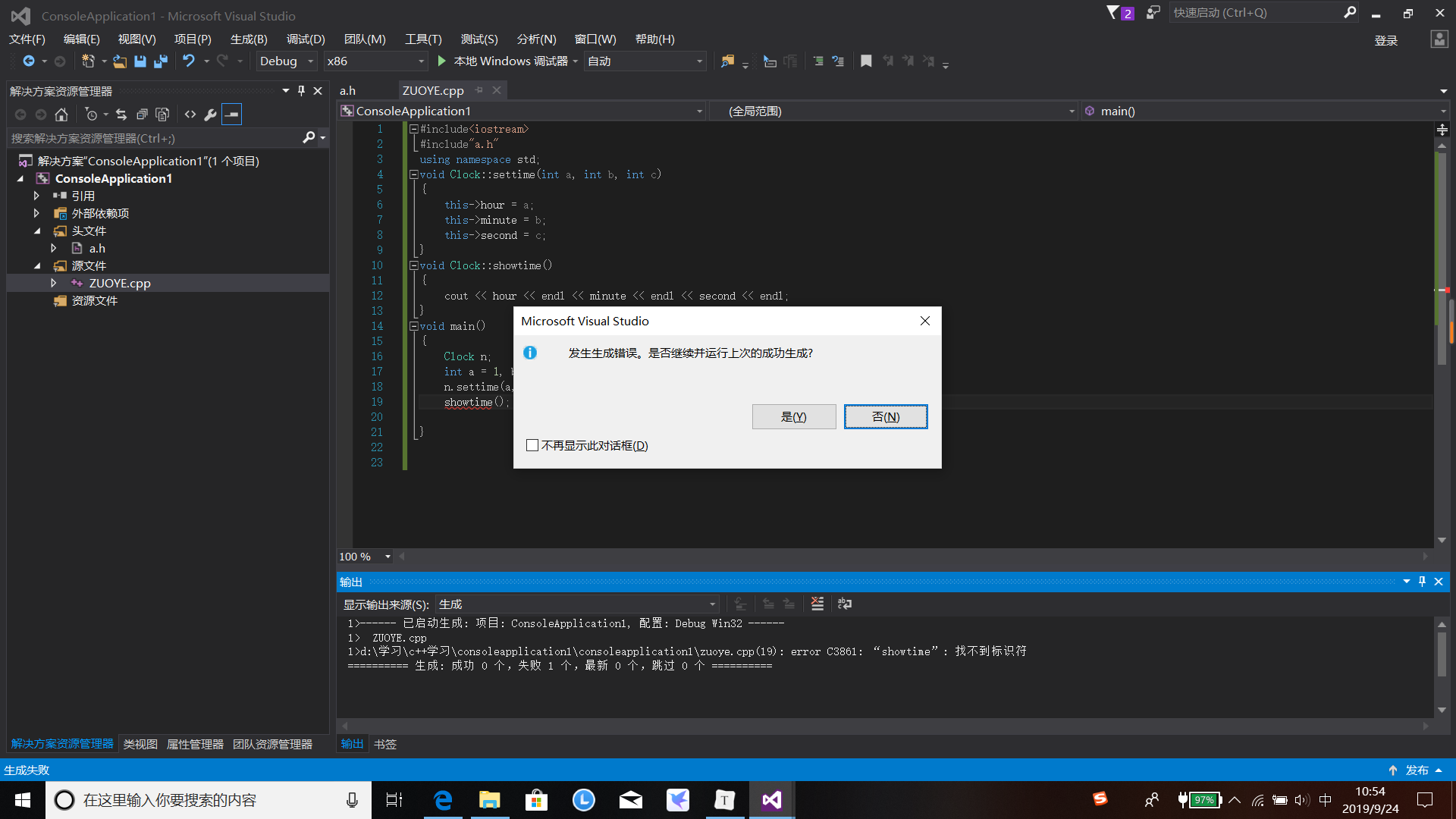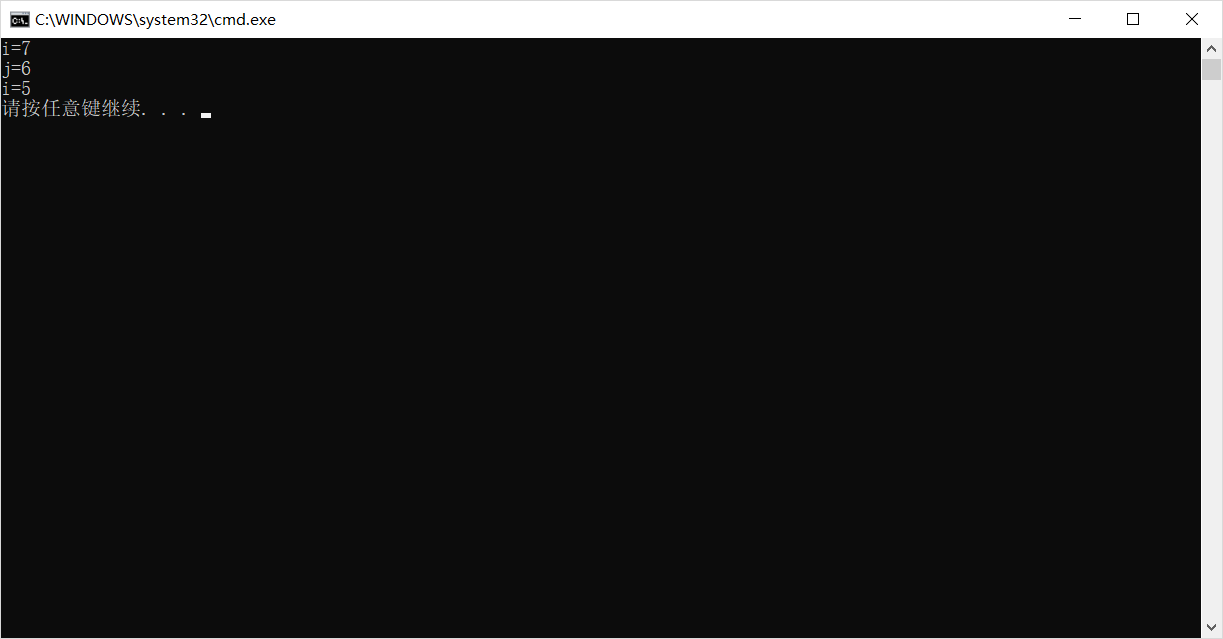# c++第三次作业----作用域

## 2：局部作用域

#include<iostream>
using namespace std;

int main()
{
int i;
int b = 3;
for (i = 0; i<1;  i++)
{
int b = 1;
cout << b << endl;
}
cout << b << endl;
}


## 3：类作用域

#ifndef a_h
#define a_h
class Clock {
int hour, minute, second;
public:
void settime(int a, int b, int c);
void showtime();

};
#endif

#include<iostream>
#include"a.h"
using namespace std;
void Clock::settime(int a, int b, int c)
{
this->hour = a;
this->minute = b;
this->second = c;
}
void Clock::showtime()
{
cout << hour << endl << minute << endl << second << endl;
}
void main()
{
Clock n;
int a = 1, b = 2, c = 3;
n.settime(a, b, c);
n.showtime();

}## 4：命名空间的作用域

#include<iostream>
using namespace std;
int i;
namespace Ns {
int j;
}
int main()
{
i = 5;
Ns::j = 6;
{
using namespace Ns;
int i;
i = 7;
cout << "i=" << i << endl;
cout << "j=" << j << endl;

}
cout << "i=" << i << endl;
return 0;
}


i=7

j=6

i=5posted @ 2019-09-24 16:47  不见风雨不见你  阅读(162)  评论(0编辑  收藏  举报